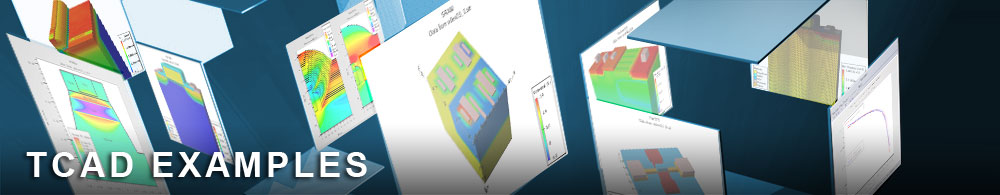PBTS(Positive Bias Temperature Stress) with Hydrogen Passivation Using Multistate Trap Model

tftex19.in : PBTS(Positive Bias Temperature Stress) with Hydrogen Passivation Using Multistate Trap Model

Requires: Victoryprocess/Atlas

Minimum Versions: Victoryprocess: 7.22.0.R/Atlas 5.28.1.R

We have shown the positive bias temperature stress simulation in tftex17.in using chemical ion transport model. In this example, we will demonstrate the Positive Bias Temperature Stress simulation due to electron trapping by multistate trap model. This model is described well in Atlas manual section of 3.3.4. It can define various trap state of the material system such as a-Si:H and IGZO(InGaZnO). In Victoryprocess part, we simulated the hydrogen diffusion in etch stopper process. The partial pressure during etch stopper process show the different hydrogen concentration. In Victorydevice, we simulate the electrochemical reaction formula for explaining the positive threshold voltage shift by electron trapping as well as hydrogen passivation forming OH-(Hydroxyl) bond.

The hydrogen atom is a donor in a-IGZO material when it forms to make a OH-(Hydroxyl) bond as well as it passivate the neutral precursor state(electron trap site: O(neutral)). This neutral precursor trap can be an electron trapping state or passivated by hydrogen atom. We simulated the hydrogen passivation reaction and electron trapping reaction using multistate trap model. For simiplicity and practical use of multistate trap model, we employed the basic 3-state. The state#1 is neutral precursor state and state#2 is negatively charged state which trap the electron, and state#3 is neutral passivated state by hydrogen. We will estimate each state's occupation probability and also the effect on the threshold voltage shift.

Victoryprcess process simulation and hydrogen diffusion

• Bottom gate SiOx etch stopper a-IGZO structure formation using Victoryprocess:

-To simulate hydrogen diffusion process, we used material/interface statement to modify a default hydrogen diffusion coefficient in Victoryprocess.
-The hydrogen concentration depending on partial pressure is adjusted in etch stopper deposition, and it diffuse in subsequent diffusion process.

Atlas Multistate Trap Simulation

After hydrogen diffusion process and structure formation, initial hydrogen concentration is imported from Victoryprocess using doping f.doping=tftex19_0.lib species1
The hydrogen is named as "species1" in the following simulation. This C-interpreter function(tftex19_0.lib) reads hydrogen profile from process structure into device structure. After reading hydrogen concentration, we define each charge state through TRAP and INTTRAP statement.
In this example, initial IGZO state can be depicted as neutral precursor state. It will decompose to negatively charged state(IGZO-) by electron trapping and neutral passivated state(IGZOH) by hydrogen passivation. The overall hydrogen passivation rate is affected by hydrogen concentration, rate of passivation, and hydrogen diffusion.
The first trap is fixed charge from ionized oxygen vacancy(Vo2+) which is not transform to other state. MSC1.FT0/MSC2.FT0/MSC3.FT0 are initial occupation probability and only state#1 is occupied with charge state +2. This state will give two free electron to the conduction band. f.extmscrates read the C-function which define the tranistion rate among states. If you look at the tftex19_1.lib file, there is no transition rate among states with *rate=0. MSC1.SP1/MSC2.SP1/MSC3.SP3 define the how many "species1 atom(ex, hydrogen) is occupied at each state. Because there is no transition rate, MSC#.SP1 have no meaning in this fixed bulk trap state. This trap statement is just to fit the initial transfer curve by somewhat initial ionized trap. It is initial Vo2+(donor-like trap). MSC.NSTATES=3 says that the total number of available state is three.(precursor/electron trapping/hydrogen passivated state) The multistate trap model is enabled with MSCTRAP option in trap/inttrap statement.

trap density=1.5e17*0.7 degen.fac=1 sign=1.0e-16 sigp=2.0e-18 msctrap msc.nstates=3 \ msc1.ft0=1.0 msc1.sp1=0 msc1.charge=2 msc1.et.cb msc1.elevel=0.2 \ msc2.ft0=0.0 msc2.sp1=1 msc2.charge=2 \ msc3.ft0=0.0 msc3.sp1=1 msc3.charge=0 msc3.elec=2 f.extmscrates=tftex19_1.lib \ x.min=-12.0 x.max=12.0 y.min=-0.245 y.max=-0.2
Next, we define the bulk trap density for various charge states. MSC1.charge=-1 is occupied with one electron which means it is negatively charged state. MSC2.charge=-2 is electron traped state which trap one more electron. This state is responsible for positve shift of threshold voltage. MSC3.charge=-1 is single negatively charged state with electron and is occuppied with species1(hydrogen) by MSC3.SP1=1. Each state's transition rate is defined in f.extmscrates="tftex19_2.lib" The state#1 can be one of possible electron trap site such as oxygen deficiency(interstitial or vacancy) or unbounded metal dangling bonds. The state#3 is the passivated state which could be hydridic passivation of metal dangling bonds. Thus, precursor state is fully acccesible into other state via non-negligible transition rate.

#bulk trap density=1.5e17*0.7 degen.fac=1 sign=1.0e-27*0.4*0.5*5 sigp=2.0e-29 msctrap msc.nstates=3 \ msc1.ft0=1.0 msc1.sp1=0 msc1.charge=-1 msc1.elec=1 \ msc2.ft0=0.0 msc2.sp1=0 msc2.charge=-2 msc2.elec=2 \ msc3.ft0=0.0 msc3.sp1=1 msc3.charge=-1 msc3.elec=1 \ f.extmscrates="tftex19_2.lib" x.min=-12.0 x.max=12.0 y.min=-0.245 y.max=-0.2 probe_id=1
We added two interface trap state using INTTRAP statement as the same way as the TRAP statements. For general ion transport model, we need to define number of species and charge state. In this example, we define hydrogen as MODELS NSPECIE=1 SPECIES1.Z=0.

For drift-diffusion simulation of hydrogen atom during applied bias and temperature, we need to define the diffusion coefficient. Other ion species is immobile. In this example, we assumed the same diffusivity over all regions.
material species1.ea=1.5 species1.af=1.5e10 species1.hop=1e-6
The probe statement measure the occupation probability of each ion speceis, concentration, and transition rate at the various point in the device during stress time.

The Vt shift due to each hydrogen concentration (low/med/high) is simulated and the results are plotted in Tonyplot.

To load and run this example, select the Load example button in DeckBuild. This will copy the input file and any support files to your current working directory. Select the run button to execute the example.